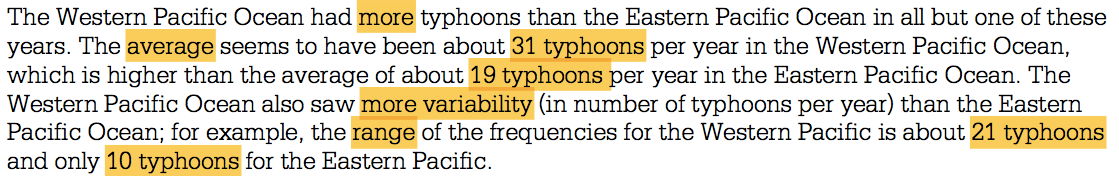# Question 1 (b)# Question 2 (c)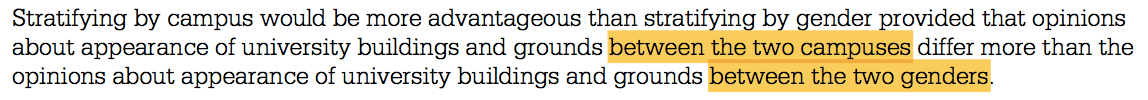# Question 4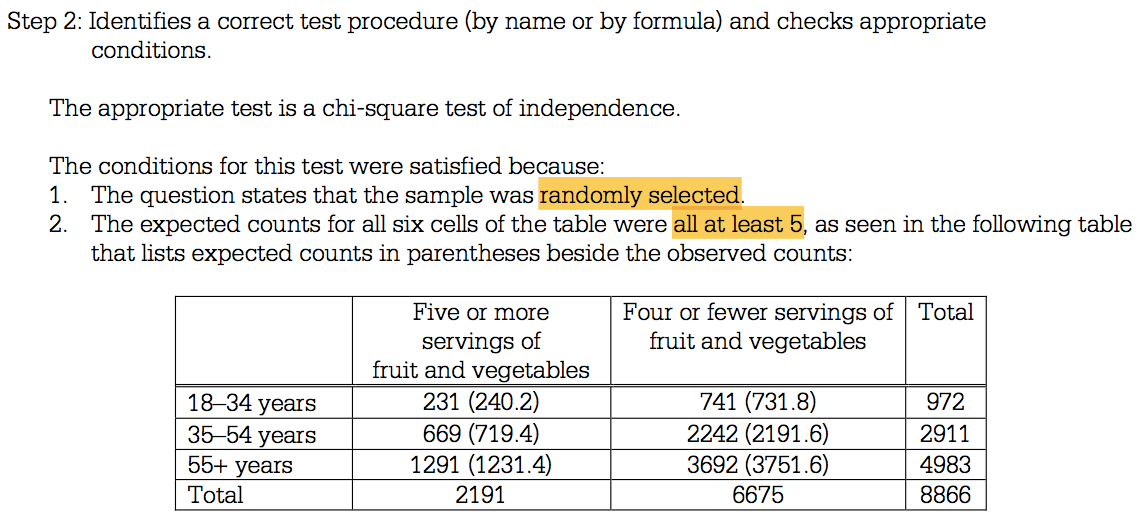Statistic/Parameter Condition/Assumption How do we check?

More two Sample Proportions

(Test for Homogeneity)

1. Count Condition: The data are counts.

2. Independent Condition: Data in groups are independent.

3. Large sample

1. Verify this.

2. SRS and 10n < N

3. Count > 5# Question 5 (a)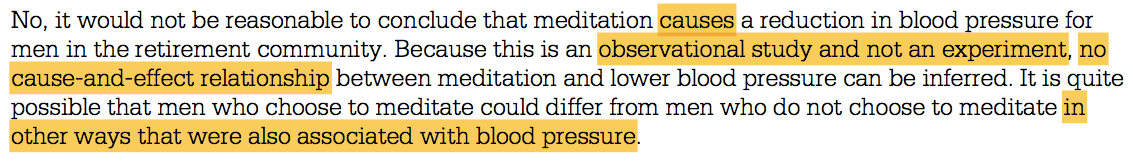# Question 5 (b)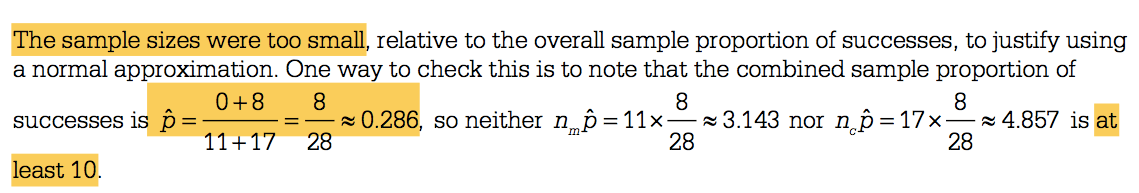Statistic/Parameter Condition/Assumption How do we check?

Two Sample Proportions

(Independent)

1. Randomization Condition: Samples in each group are random samples (SRS) or representatives of their populations or in experiments the treatments are randomly assigned.

2. Normality Condition: n1p1 and n2p2 ≥ 10 and n1q1 and n2q2 ≥ 10.

3. Independent Condition: The selection of each subject is independent of each other (10n < N) for each sample. In some experiments this is not necessary.

4. Independence of Groups Condition: The groups are independent of each other.

1. Based on the information provided.

2. Show that the inequalities are true.

3. Show that the inequality is true.

4. Based on the information provided.

# Question 5 (c)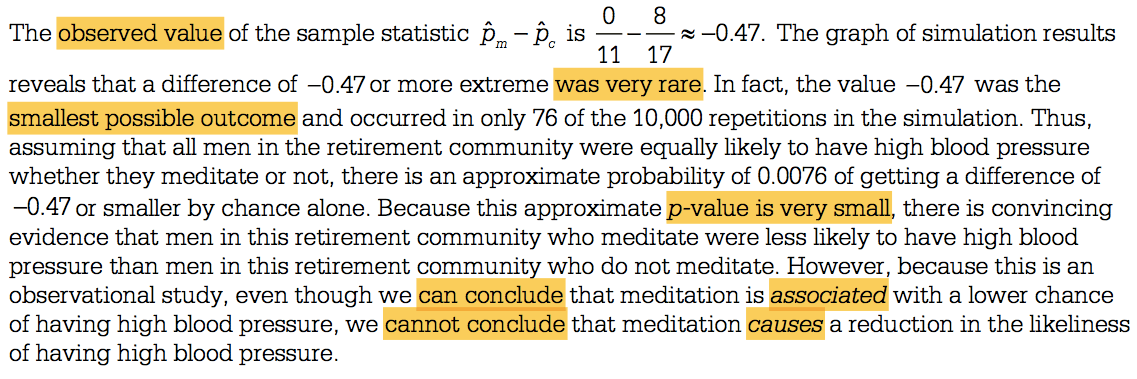# Question 6 (a)